# Getting Started with canvasXpress in R

## Examples

### Scatter 3D Plot

y <- read.table("https://canvasxpress.org/data/cX-irist-dat.txt", header=TRUE, sep="\t",
quote="", row.names=1, fill=TRUE, check.names=FALSE, stringsAsFactors=FALSE)
quote="", row.names=1, fill=TRUE, check.names=FALSE, stringsAsFactors=FALSE)

canvasXpress(data      = y,
varAnnot  = z,
graphType ="Scatter3D",
colorBy   = "Species",
ellipseBy = "Species",
xAxis     = list("Sepal.Length"),
yAxis     = list("Petal.Width"),
zAxis     = list("Petal.Length"),
theme     = "CanvasXpress",
title     = "Iris Data Set",
axisTickScaleFontFactor  = 0.5,
axisTitleScaleFontFactor = 0.5)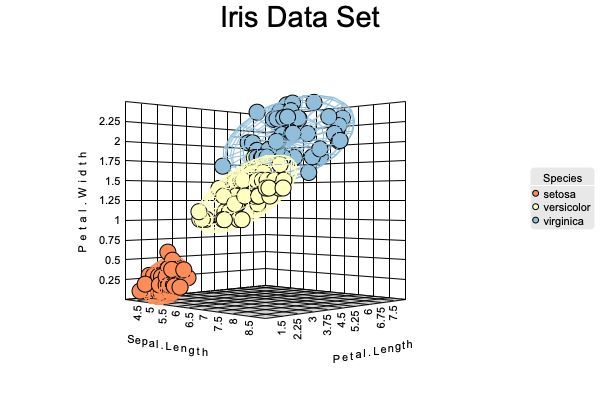Scatter3D

### Scatter 2D Matrix Plot

y <- read.table("https://canvasxpress.org/data/cX-irist-dat.txt", header=TRUE, sep="\t",
quote="", row.names=1, fill=TRUE, check.names=FALSE, stringsAsFactors=FALSE)
quote="", row.names=1, fill=TRUE, check.names=FALSE, stringsAsFactors=FALSE)

canvasXpress(data              = y,
varAnnot          = z,
graphType         = "Scatter2D",
colorBy           = "Species",
scatterPlotMatrix = TRUE,
theme             = "CanvasXpress")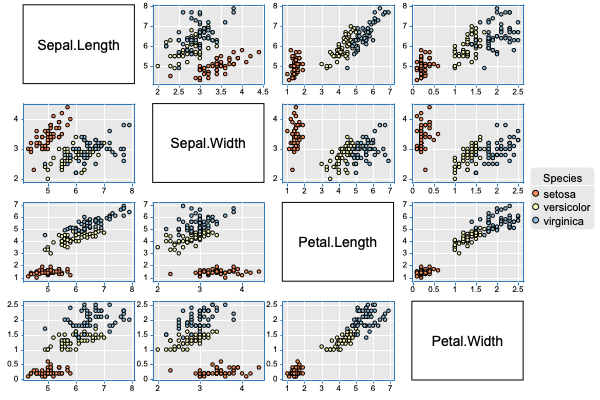Scatter2DMatrix

### Boxplot

y <- read.table("https://canvasxpress.org/data/cX-iris-dat.txt", header=TRUE, sep="\t",
quote="", row.names=1, fill=TRUE, check.names=FALSE, stringsAsFactors=FALSE)
quote="", row.names=1, fill=TRUE, check.names=FALSE, stringsAsFactors=FALSE)

canvasXpress(data                  = y,
smpAnnot              = x,
graphType             = "Boxplot",
groupingFactors       = list("dose", "supp"),
stringSampleFactors   = list("dose"),
graphOrientation      = "vertical",
colorBy               = "dose",
title                 = "The Effect of Vitamin C on Tooth Growth in Guinea Pigs",
smpTitle              = "dose",
xAxisTitle            = "len",
smpLabelRotate        = 90,
xAxisMinorTicks       = FALSE,
xAxis2Show            = FALSE,
legendScaleFontFactor = 1.8)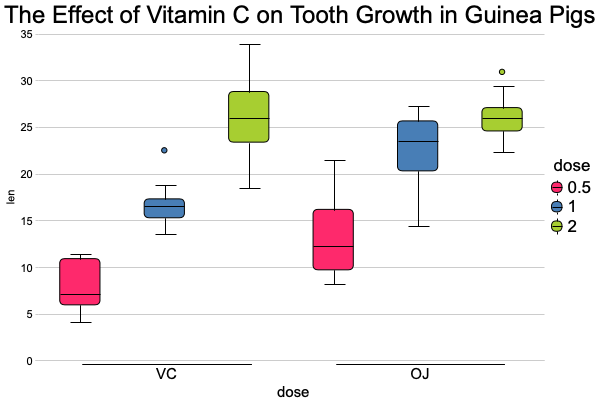Boxplot

### Heatmap

y  <- read.table("https://canvasxpress.org/data/cX-multidimensionalheatmap-dat.txt",
check.names=FALSE, stringsAsFactors=FALSE)
check.names=FALSE, stringsAsFactors=FALSE)
check.names=FALSE, stringsAsFactors=FALSE)
check.names=FALSE, stringsAsFactors=FALSE)
header=TRUE, sep= "\t", quote="", row.names=1, fill=TRUE,
check.names=FALSE, stringsAsFactors=FALSE)
header=TRUE, sep= "\t", quote="", row.names=1, fill=TRUE,
check.names=FALSE, stringsAsFactors=FALSE)

canvasXpress(data                 = list(y = y, data2 = y2, data3 = y3, data4 = y4),
smpAnnot             = x,
varAnnot             = z,
graphType            = "Heatmap",
guides               = TRUE,
outlineBy            = "Outline",
outlineByData        = "data2",
shapeBy              = "Shape",
shapeByData          = "data3",
sizeBy               = "Size",
sizeByData           = "data4",
showHeatmapIndicator = FALSE,
afterRender          = list(list("clusterSamples")))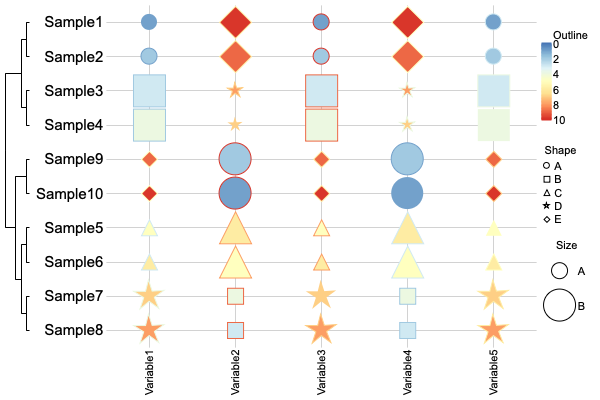Heatmap

### Four-way Venn Diagram

canvasXpress(vennData   = data.frame(AC=456, A=340, ABC=552, ABCD=148, BC=915,
ACD=298, BCD=613, B=562, CD=143, ABD=578,
graphType  = "Venn",
vennLegend = list(A="List 1", D="List 4", C="List 3", B="List 2"),
vennGroups = 4)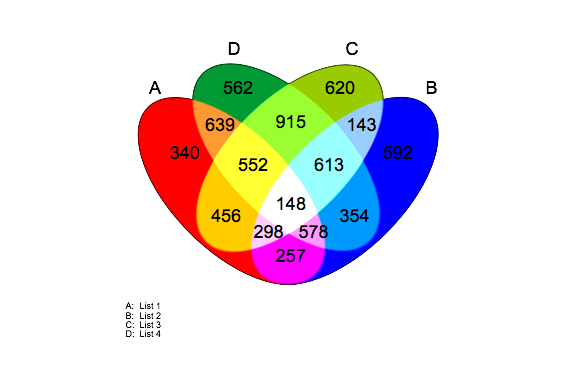Venn

#List example names
cxShinyExample(example = "example1")